RS Aggarwal Class 8 Solutions Chapter 14 - Polygons

RS Aggarwal Class 8 Chapter 14 - Polygons Solutions Free PDF

A polygon is a two-dimensional shape which is formed by a finite chain of straight line segments closes in a loop to form a closed circuit. In this chapter, the students will learn the concepts of a polygon, convex polyhedrons and regular polyhedrons. RS Aggarwal class 8 solutions chapter 14 polygons are provided here so that students can go through the solutions while facing difficulties in solving the RS Aggarwal questions.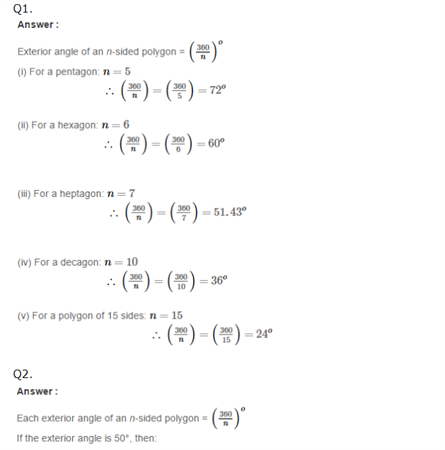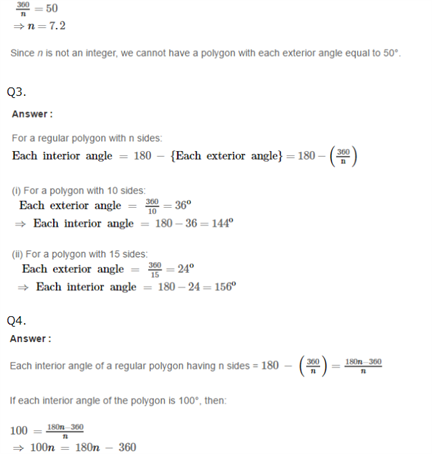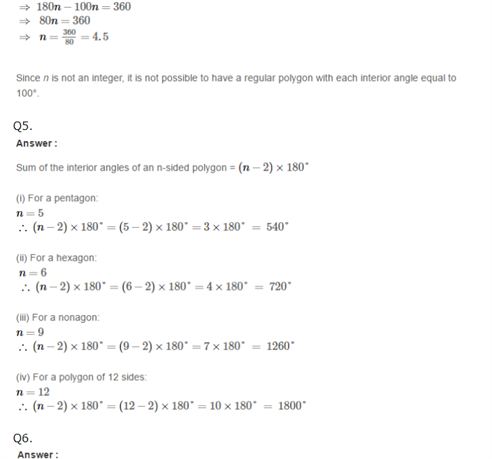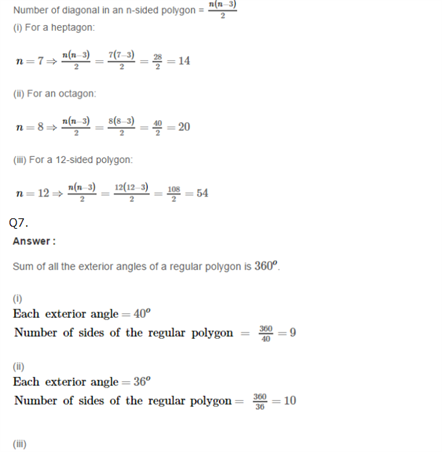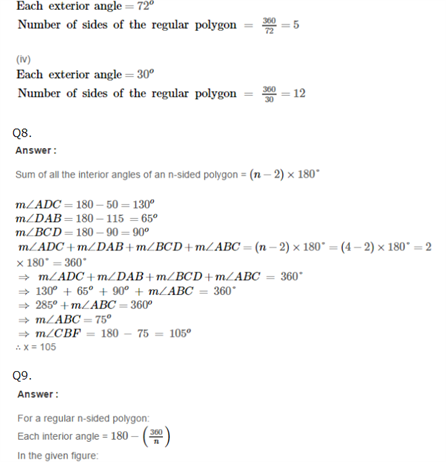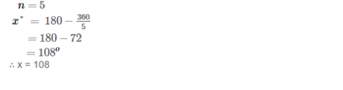Practise This Question

If Swamy has two children and he truthfully answers yes to the question “Is at least one of your children a girl?”, what is the probability that both his children are girls?